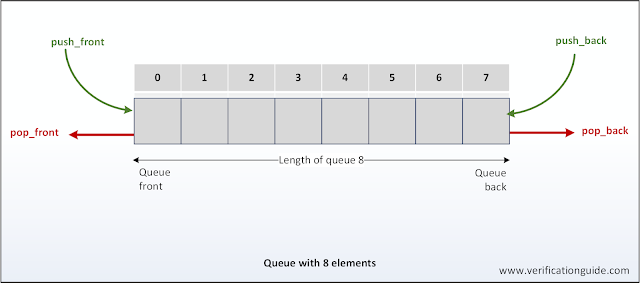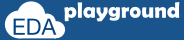# Queue in SystemVerilog

A queue is a variable-size, ordered collection of homogeneous elements.
• like a dynamic array, queues can grow and shrink
• queue supports adding and removing elements anywhere.

Queues are declared using the same syntax as unpacked arrays, but specifying \$ as the array size. In queue 0 represents the first, and \$ representing the last entries.

A queue can be bounded or unbounded.
• bounded queue     - queue with the number of entries limited or queue size specified
• unbounded queue - queue with unlimited entries or queue size not specified

## Queue Declaration:

data_type queue_name[\$];
where:
data_type        -  data type of the queue elements.
queue_name    -  name of the queue.

### Queue Declaration Example:

```   bit    queue_1[\$];     // queue of bits  (unbound queue)
int    queue_2[\$];     // queue of int
byte   queue_3[\$:255]; // queue of byte (bounded queue with 256 entries)
string queue_4[\$];     // queue of strings
```

## Queue Initialization:

```   queue_1  = {0,1,2,3};
queue_4  = {“Red”,"Blue”,"Green”};
```

### Unbounded QueueSystemVerilog queue

### Bounded QueueBounded queue

## Queue Methods:

size()            -->    returns the number of items in the queue.
insert()          -->    inserts the given item at the specified index position.
delete()         -->    deletes the item at the specified index position.
push_front()  -->    inserts the given element at the front of the queue.
push_back() -->    inserts the given element at the end of the queue.
pop_front()   -->    removes and returns the first element of the queue.
pop_back()   -->    removes and returns the last element of the queue.

### Unbounded Queue Declaration, Initialization, Size, Insert and Delete Method

This example shows the declaration and usage Queue methods.

```module queues_array;
//declaration
bit    [31:0] queue_1[\$]; //unbounded queue
string  queue_2[\$];

initial begin
//Queue Initialization:
queue_1 = {0,1,2,3};
queue_2 = {"Red","Blue","Green"};

//Size-Method
\$display("----- Queue_1 size is %0d  -----",queue_1.size());
foreach(queue_1[i]) \$display("\tqueue_1[%0d] = %0d",i,queue_1[i]);
\$display("----- Queue_2 size is %0d  -----",queue_2.size());
foreach(queue_2[i]) \$display("\tqueue_2[%0d] = %0s",i,queue_2[i]);

//Insert-Method
queue_2.insert(1,"Orange");
\$display("----- Queue_2 size  after inserting Orange is %0d  -----",queue_2.size());
foreach(queue_2[i]) \$display("\tqueue_2[%0d] = %0s",i,queue_2[i]);

//Delete Method
queue_2.delete(3);
\$display("----- Queue_2 size after Delete is %0d  -----",queue_2.size());
foreach(queue_2[i])\$display("\tqueue_2[%0d] = %0s",i,queue_2[i]);
end

endmodule```

#### Simulator Output

----- Queue_1 size is 4 -----
queue_1 = 0
queue_1 = 1
queue_1 = 2
queue_1 = 3
----- Queue_2 size is 3 -----
queue_2 = Red
queue_2 = Blue
queue_2 = Green
----- Queue_2 size after inserting Orange is 4 -----
queue_2 = Red
queue_2 = Orange
queue_2 = Blue
queue_2 = Green
----- Queue_2 size after Delete is 3 -----
queue_2 = Red
queue_2 = Orange
queue_2 = Blue
Execute the above code on### Queue, push_front(), push_back(), pop_front() and pop_back() MethodQueue Operations

```module queues_array;
//declaration
bit    [31:0] queue_1[\$];
int    lvar;

initial begin
//Queue Initialization:
queue_1 = {0,1,2,3};

//Size-Method
\$display("\tQueue_1 size is %0d",queue_1.size());

//Push_front Method
queue_1.push_front(22);
\$display("\tQueue_1 size after push_front is %0d",queue_1.size());

//Push_back  Method
queue_1.push_back(44);
\$display("\tQueue_1 size after push_back is %0d",queue_1.size());

//Pop_front Method
lvar = queue_1.pop_front();
\$display("\tQueue_1 pop_front value is %0d",lvar);

//Pop_back Method
lvar = queue_1.pop_back();
\$display("\tQueue_1 pop_back value is %0d",lvar);
end
endmodule
```

#### Simulator Output

Queue_1 size is 4
Queue_1 size after push_front is 5
Queue_1 size after push_back is 6
Queue_1 pop_front value is 22
Queue_1 pop_back value is 44

### Bounded queue declaration and accessing

The number of entries of the bounded queue is limited, push_back to the bounded queue (after the queue full condition) will not impact any changes to the queue.  push_front to the bounded queue (after the queue full condition) will delete the last entry from queue and stores a new entry in the 0th index of the queue.Bounded Queue Operations

```module queues_array;
//declaration
int    queue[\$:2];
int    index;
int  temp_var;

initial begin
//Queue Initialization:
queue = {7,3,1};

\$display("Queue elements are,");
\$display("\tqueue = %p",queue);

queue.push_back(10);

\$display("After push_back Queue elements are,");
\$display("\tqueue = %p",queue);

queue.push_front(10);

\$display("After push_front Queue elements are,");
\$display("\tqueue = %p",queue);
end

endmodule
```

#### Simulator Output

Queue elements are,
queue = '{7, 3, 1}
After push_back Queue elements are,
queue = '{7, 3, 1}
After push_front Queue elements are,
queue = '{10, 7, 3}

### Accessing random element of queue

In the below example, random queue entry will be accessed by using index. Unlike pop_front/pop_back option queue entry will not get deleted on accessing with an index of the queue.

```module queues_array;
//declaration
int    queue[\$];
int    index;
int  temp_var;

initial begin
//Queue Initialization:
queue = {7,3,1,0,8};

\$display("----- Queue elements with index -----");
foreach(queue[i])
\$display("\tqueue[%0d] = %0d",i,queue[i]);
\$display("-------------------------------------\n");

\$display("Before Queue size is %0d",queue.size());
repeat(2) begin //{
index    = \$urandom_range(0,4); //index of queue is from 0 to 4
temp_var = queue[index];
\$display("Value of Index %0d in Queue is %0d",index,temp_var);
end //}
\$display("After Queue size is %0d",queue.size());
end
endmodule
```

#### Simulator Output

----- Queue elements with index -----
queue = 7
queue = 3
queue = 1
queue = 0
queue = 8
-------------------------------------

Before Queue size is 5
Value of Index 3 in Queue is 0
Value of Index 0 in Queue is 7
After Queue size is 5

### Deleting random element of queue with index

Calling queue.delete(index) method will delete the entry stored with 'index'.

```module queues;
//declaration
int    queue[\$];
int    index;
int  temp_var;

initial begin
//Queue Initialization:
queue = {7,3,1,0,8};

\$display("Queue entries are %p",queue);
\$display("Before Queue size is %0d",queue.size());
index    = \$urandom_range(0,4); //index of queue is from 0 to 4
\$display("Index %0d is deleted",index);
queue.delete(index);
\$display("After Queue size is %0d",queue.size());
\$display("Queue entries are %p",queue);

\$display("\nQueue entries are %p",queue);
\$display("Before Queue size is %0d",queue.size());
index    = \$urandom_range(0,3); //index of queue is from 0 to 4
\$display("Index %0d is deleted",index);
queue.delete(index);
\$display("After Queue size is %0d",queue.size());
\$display("Queue entries are %p",queue);
end
endmodule
```

#### Simulator Output

Queue entries are '{7, 3, 1, 0, 8}
Before Queue size is 5
Index 3 is deleted
After Queue size is 4
Queue entries are '{7, 3, 1, 8}

Queue entries are '{7, 3, 1, 8}
Before Queue size is 4
Index 0 is deleted
After Queue size is 3
Queue entries are '{3, 1, 8}

### Deleting complete queue

Calling queue.delete() method will delete the complete queue, which leads to the deletion of all the entries of the queue.

```module qu_delete;
//queue declaration
int qu[\$];

initial begin

qu.push_back(2);
qu.push_back(13);
qu.push_back(5);
qu.push_back(65);

\$display("[Before-Delete] Queue size is %0d",qu.size());
qu.delete();
\$display("[After -Delete] Queue size is %0d",qu.size());

end
endmodule
```

#### Simulator Output

[Before-Delete] Queue size is 4
[After -Delete] Queue size is 0